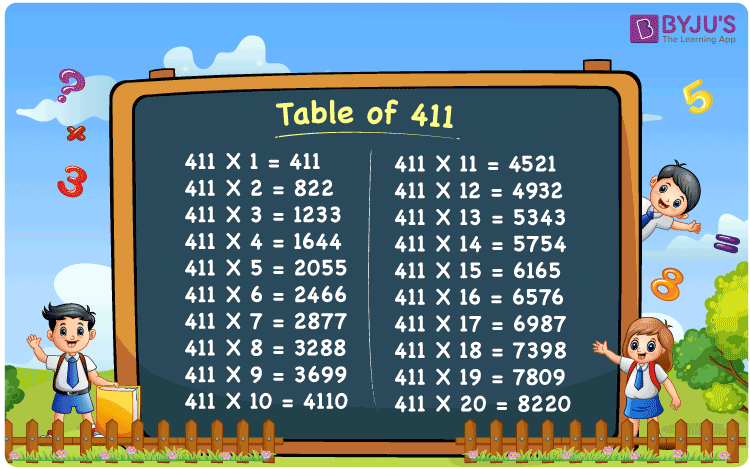Checkout JEE MAINS 2022 Question Paper Analysis : Checkout JEE MAINS 2022 Question Paper Analysis :

# Table of 411

The table of 411 is the multiplication table for the number four hundred and eleven, that can be created using the multiplication by natural numbers. In mathematics, multiplication tables are generated through multiplication and repeated addition techniques. Thus, we can write the table of 411 either by multiplication or by repeated addition. In this article, you will learn how to create the multiplication table up to 20 times with the help of a chart and an example.

## Table of 411 Chart## What is the 411 Times Table?

The 411 times table is the table of 411 that is formed through the multiplication by natural numbers and addition of 411 by itself. The below table shows the 411 times table up to 10 times.

 Using multiplication Using repeated addition 411 × 1 = 411 411 411 × 2 = 822 411 + 411 = 822 411 × 3 = 1233 411 + 411 + 411 = 1233 411 × 4 = 1644 411 + 411 + 411 + 411 = 1644 411 × 5 = 2055 411 + 411 + 411 + 411 + 411 = 2055 411 × 6 = 2466 411 + 411 + 411 + 411 + 411 + 411 = 2466 411 × 7 = 2877 411 + 411 + 411 + 411 + 411 + 411 + 411 = 2877 411 × 8 = 3288 411 + 411 + 411 + 411 + 411 + 411 + 411 + 411 = 3288 411 × 9 = 3699 411 + 411 + 411 + 411 + 411 + 411 + 411 + 411 + 411 = 3699 411 × 10 = 4110 411 + 411 + 411 + 411 + 411 + 411 + 411 + 411 + 411 + 411 = 4110

## Multiplication Table of 411

Learn the multiplication table of 411 using the simple multiplication of 411 by numbers from 1 to 20 here.

 411 × 1 = 411 411 × 2 = 822 411 × 3 = 1233 411 × 4 = 1644 411 × 5 = 2055 411 × 6 = 2466 411 × 7 = 2877 411 × 8 = 3288 411 × 9 = 3699 411 × 10 = 4110 411 × 11 = 4521 411 × 12 = 4932 411 × 13 = 5343 411 × 14 = 5754 411 × 15 = 6165 411 × 16 = 6576 411 × 17 = 6987 411 × 18 = 7398 411 × 19 = 7809 411 × 20 = 8220

## Example on the Table of 411

Question:

The capacity of a water tank is 411 litres. How much water can be stored in 14 such tanks?

Solution:

Given,

The capacity of a water tank = 411 litres

Number of tanks = 14

Total quantity of water that can be stored in 14 tanks = ?

Using the table of 411, 411 × 14 = 5754

Hence, we can store 5754 litres of water in 14 water tanks of the given capacity.

## Frequently Asked Questions on Table of 411

### What is the table of 411?

The table of 411 is obtained when we multiply the numbers 411 by 1, 2, 3, and so on. Some of the entries of the 411 times table are given below:
411 × 1 = 411
411 × 2 = 822
411 × 3 = 1233
411 × 4 = 1644
411 × 5 = 2055

### Is it possible to generate the 411 times table using addition?

Yes, it is possible to generate the 411 times table using addition. For this, we should add the number 411 repeatedly as many times as specified. For example, 411 times 4 can be calculated by addition 411 for four times, i.e. 411 times 4 = 411 + 411 + 411 + 411 = 1644.

### Find the value of 411 times 19.

Using the table of 411, 411 times 19 = 411 × 19 = 7809.